## Welcome to String Wizard

String Wizard is a JavaScript calculator designed especially for wire strings. It can of course be used for calculations relating to any kind of stringed instrument, as ‘the physics’ are the same for all types of strings...in this universe! Its main purpose however, is to help players and makers of metal–strung harps reduce the amount of practical experimentation necessary in order to explore different stringing options for their instruments by providing a guide as to what might be expected when manipulating different parameters of a string.

It must be said at the outset that ultimately a final decision regarding stringing should always be arrived at based on experiment. The instrument itself is not factored into any of the calculations, so a string that doesn’t quite work out on paper might sound just fine in the real world and vice versa.

The calculator is divided into five sections.

### Section 1 – Common Parameters

The first section deals with the more common or readily observed parameters of a string, those associated with the well–known Mersenne–Taylor equation: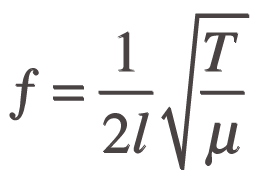Where

ƒ = frequency
l = length
T = tension
µ = linear density (mass per unit length)

The linear density or mass per unit length µ is a function of the density ? of the material and the diameter d of the string.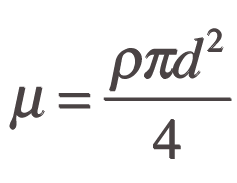Using the known parameters of a string and different inversions of the equation, the calculator can work backwards to solve for the value or a possible range of values for other parameters.

### Section 2 – Strength

The second section of the calculator deals with the strength of the material and provides a guideline for the optimum tension on a particular string. The values returned in the orange boxes in this section represent the practicable range of tension for wire of a given strength. This is simply based on the assumption of a maximum safety factor  of three semitones below the elastic limit of the string, calculated from the string’s diameter and the yield strength of the material as entered in the relevant fields. Data for the yield strength of commonly used modern alloys as presented in Table 1 have been gathered from a variety of sources and probably represent an average for each metal. The elastic limit of a particular ‘batch’ of wire is best determined by experiment (see example 4 in the ‘possible applications’ page for the calculator). The ‘sweet spot’ for a particular string may also be determined in the same way.

### Section 3 – Inharmonicity

The third section calculates the coefficient of inharmonicity for the string based on parameters entered in the first section (length l, diameter d, density ? and frequency ƒ) and the material’s modulus of elasticity Q according to the equations given by Robert W. Young in ‘Inharmonicity of Plain Wire Piano Strings’ (1952).

The coefficient of inharmonicity is given both in ‘Hz’ and ‘cents’  in the first two orange boxes. For calculations in Hertz the coefficient of inharmonicity corresponds to: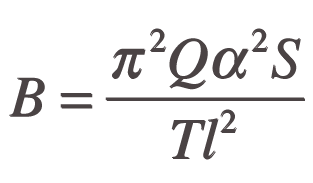Where S is the cross–sectional area of the string and a the radius of gyration. The equation can be rewritten using the parameters listed above.

Some authors (including Young) use a different definition of the coefficient of inharmonicity (corresponding to B*865.62) to calculate the inharmonicity d of a given partial in cents, where n is the partial number and B is the coefficient of inharmonicity (in cents).

d = Bn2

Both methods yield results within less than 1% of each other where inharmonicity is over 30 cents.

The two lower orange boxes return the frequency of a partial and the amount of deviation in cents, based on the coefficient of inharmonicity in Hertz, and the partial number entered in the field labelled ‘Partial No.’ and the fundamental frequency ƒ. The partial frequencies estimated by means of these equations have been found to be in good agreement with those measured in real strings but, as with the other calculations, the instrument itself is has not been factored in. The equations assume that the strings are anchored at both ends to perfectly static points and do not take account of the fact that the limbs of the harp and its soundboard are not perfectly rigid. As with the other aspects of string tones discussed here, the acceptable level of inharmonicity for a particular harp string will be best determined by experiment.

### Section 4 – Lateral Resistance

The fourth section of the calculator deals with what might be termed the ‘lateral resistance’ of the string or its ‘playability’. This basically relates to how the string feels to the player. For example, a string that offers relatively little resistance might be described as weak or floppy while one that offers a lot of resistance could be described as tight.

Contrary to what our instincts might tell us, the ‘relative strength’ or elasticity of the material contributes very little to this. As long as the string is under enough tension to vibrate, the force pulling on the string at both ends (the tension on the string) will counteract the force needed to displace the string sideways by a certain amount to pluck it. On the other hand, the elasticity of the material affects the amount of energy stored in the string and this makes the world of difference to what happens once the string is plucked. Based on the relevant parameters the field labelled ‘Elastic Potential Energy’ returns a value for the amount of energy stored in the string at the moment it is being plucked.

The parameters that do have a bearing on the string’s lateral resistance are tension T, length l, the point on the string where it is being plucked, and the ‘depth’ of the plucking action (how far the string travels before it is released).

The equation describing the relationship between these parameters is complex and including it in this calculator would be well and truly beyond this JavaScript programmer’s skills. However, because the plucking distance will always represent a very small fraction of the overall length of a string it is possible to use some approximations to formulate an equation that returns a value for the amount of force necessary to displace a string sideways by a certain amount within a ±1% margin of error. The ‘lateral resistance’ of a string could be expressed as the negative equivalent of the force required to pluck the string Fp.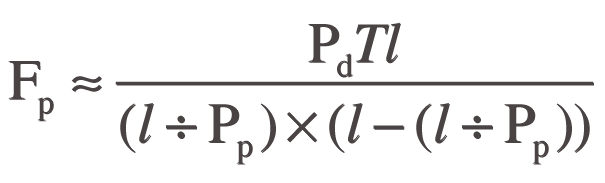Where

Pp = plucking point
Pd = plucking depth

The value entered in the field labelled ‘Plucking Point’ should represent the denominator of the fraction of the length of the string where it is being plucked e.g. 2 for halfway 3 for one third of the way and so on. In general bass strings will be plucked closer to the soundboard between one third and one quarter of the way up and treble strings closer to midway.

The value entered for ‘Plucking Depth’ determines the net amount based on the diameter of the string. A fixed amount here would not give an accurate picture, plucking a bass string 3mm ‘deep’ might be considered light whereas the same amount on the top string could be excessive. A side effect of non–Pythagorean string scales typically employed in the design of most metal-strung harps is that the lower strings tend to offer comparatively less resistance. 

How much lateral resistance is ideal? Is there an optimum point where the string should be plucked? How far the string should be plucked will obviously depend on how loud we want to the note to sound but the rest will be subjective and best determined by experiment.

### Section 5 – Compare String Data

The fifth section allows the user to save string data in .csv format (comma separated values) and compare different configurations. This data can be exported to a spreadsheet programme such as Microsoft Excel or Apple’s Numbers.

### Last but not least, a word of advice from the author:

If it works DON’T fix it and always consult your harp maker before changing to a different string material or a different tuning if you are unsure about anything. Enjoy.

 R. W. Young, ‘Inharmonicity of Plain Wire PianoStrings’, Journal of the Acoustical Society of America, Vol.24, no 3,(1952).

 Meaning that the relevant equations will yield results in Hertz or cents.

 Young (1952) Eq. 6.

 Young (1952) Eq. 8, S and a can be expressed in terms of d and T in terms of ƒ, l, ? and d. See also ‘Inharmonicity’.

 Young (1952) Eq. 7.

 The ‘relative strength’ or ‘net elasticity’ of a wire string is given by the modulus of elasticity of the material multiplied by the cross-sectional area of the wire: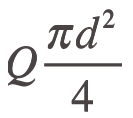A more elastic material should feel comparatively weak but ultimately, a string’s lateral resistance is determined by the amount of tension it is under. Although for a fixed pitch the string diameter will have a bearing on both strength and tension, the additional tension and the elongation resulting from the plucking action only represent a very small fraction of the overall tension on the string and the total elongation.

 Meaning that the strings less than double their lengths of every descending octave.

 If strictly Pythagorean proportions were to be maintained on a 29–string harp with a shortest string at 68 mm in length and 0.40 mm in diameter (similar to the surviving low–headed harps), the lowest string (#29) would need to be 1.08 metres long and 1.6mm in diameter for its proportions to be the same. For the lowest string to sound a low G over 80 kgs worth of tension would be required. Of course the harp would also need to be a lot stronger and heavier to be able to withstand that much tension.

 To copy the data for export in Firefox hold down the option key and drag across the text to select, for IE and Safari drag select asnormal. Copy and Paste the data into a text editor and save as .csv. This file can then be opened in most spreadsheet programmes.

Submitted by Paul Dooley, 21 March, 2013. © 2004-2013 Paul Dooley